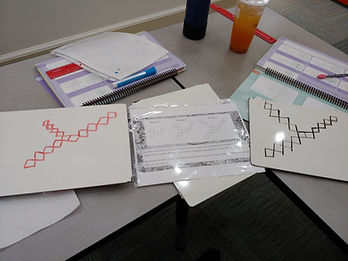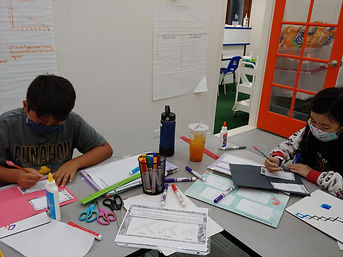## Ms. Mari

### Target 1​

###### Lesson Type:

Review

Algebra

:

Variable

Write a linear equation in slope-intercept form.

###### 1:

Use rise/run as a ratio to calculate the slope.

###### 2:

Find the slope of a line on a graph.

###### 3:

Define the type of slope depicted in a linear equation as positive, negative, zero, and undefined.

7th

###### Vocabulary:

SLOPE, RISE, RUN, RATIO, POSITIVE SLOPE, NEGATIVE SLOPE, ZERO SLOPE, UNDEFINED SLOPE

Activities:

- Completed "Making Slopes" Activity from last week -- given an ordered pair, students had to find another ordered pair to create a given slope: positive, negative, zero, undefined, fractional### Home Exploration

###### Guiding Questions:## Absent Students:

Jacob, Cameron

### Target 2

:

###### 1:

Identify and complete a growing pattern, where something new is added to each segment.

7th

###### Vocabulary:

GROWING PATTERN

Activities:

- Extended a growing pattern by drawing the next two figures.

- Determined a rule for predicting other figure numbers in this pattern.### Home Exploration

###### Guiding Questions:### Target 3

:

###### 1:

Define the slope as a rate of change.

###### 2:

Recognize m as the slope.

###### 3:

Recognize b as the y-intercept.

8th

###### Vocabulary:

SLOPE, Y-INTERCEPT, SLOPE-INTERCEPT FORM

Activities:

- Used a graphic organizer to write a description and create an equation, a table, and a graph for the growing pattern.

- Identified the slope in each representation and determined its meaning for this pattern.

- Identified the y-intercept in each representation and determined its meaning for this pattern.### Home Exploration